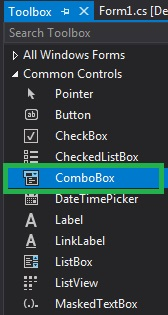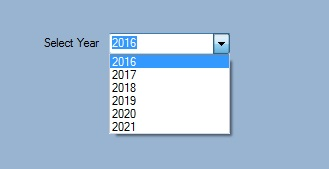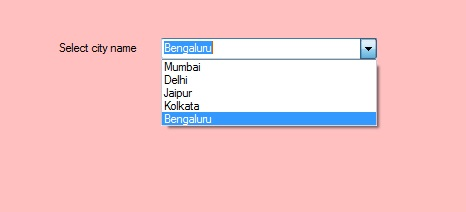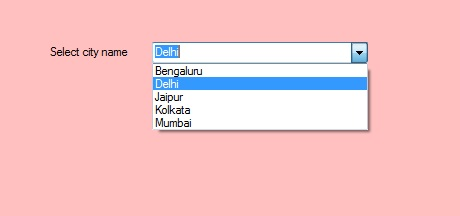Related Articles
How to Sort the Elements of the ComboBox in C#?
• Last Updated : 27 Jun, 2019

In Windows forms, ComboBox provides two different features in a single control, it means ComboBox works as both TextBox and ListBox. In ComboBox, only one item is displayed at a time and the rest of the items are present in the drop-down menu. You are allowed to sort the elements present in the ComboBox by using the Sorted Property.
If you want to sort the elements of the ComboBox, then set the value of this property to true, otherwise, false. The default value of this property is false. This sorting is case-insensitive and the elements are sort in ascending order. You can set this property using two different methods:

1. Design-Time: It is the easiest method to sort the elements present in the ComboBox control using the following steps:

• Step 1: Create a windows form as shown in the below image:
Visual Studio -> File -> New -> Project -> WindowsFormApp• Step 2: Drag the ComboBox control from the ToolBox and Drop it on the windows form. You are allowed to place a ComboBox control anywhere on the windows form according to your need.• Step 3: After drag and drop you will go to the properties of the ComboBox control to sort the elements present in the ComboBox.Output:2. Run-Time: It is a little bit trickier than the above method. In this method, you can sort the elements present in the ComboBox programmatically with the help of given syntax:

`public bool Sorted { get; set; }`

Here, the value of this property is of System.Boolean type. Following steps are used to sort the ComboBox elements:

• Step 1: Create a combobox using the ComboBox() constructor is provided by the ComboBox class.
```// Creating ComboBox using ComboBox class
ComboBox mybox = new ComboBox();
```
• Step 2: After creating ComboBox, sort the elements of the ComboBox.
```// Sort the elements of the ComboBox
mybox.Sorted = true;
```
• Step 3: And last add this combobox control to form using Add() method.
```// Add this ComboBox to form
```

Example:

 `using` `System; ` `using` `System.Collections.Generic; ` `using` `System.ComponentModel; ` `using` `System.Data; ` `using` `System.Drawing; ` `using` `System.Linq; ` `using` `System.Text; ` `using` `System.Threading.Tasks; ` `using` `System.Windows.Forms; ` ` `  `namespace` `WindowsFormsApp11 { ` ` `  `public` `partial` `class` `Form1 : Form { ` ` `  `    ``public` `Form1() ` `    ``{ ` `        ``InitializeComponent(); ` `    ``} ` ` `  `    ``private` `void` `Form1_Load(``object` `sender, EventArgs e) ` `    ``{ ` `        ``// Creating and setting the properties of label ` `        ``Label l = ``new` `Label(); ` `        ``l.Location = ``new` `Point(222, 80); ` `        ``l.Size = ``new` `Size(99, 18); ` `        ``l.Text = ``"Select city name"``; ` ` `  `        ``// Adding this label to the form ` `        ``this``.Controls.Add(l); ` ` `  `        ``// Creating and setting the properties of comboBox ` `        ``ComboBox mybox = ``new` `ComboBox(); ` `        ``mybox.Location = ``new` `Point(327, 77); ` `        ``mybox.Size = ``new` `Size(216, 26); ` `        ``mybox.Sorted = ``true``; ` `        ``mybox.Name = ``"My_Cobo_Box"``; ` `        ``mybox.Items.Add(``"Mumbai"``); ` `        ``mybox.Items.Add(``"Delhi"``); ` `        ``mybox.Items.Add(``"Jaipur"``); ` `        ``mybox.Items.Add(``"Kolkata"``); ` `        ``mybox.Items.Add(``"Bengaluru"``); ` ` `  `        ``// Adding this ComboBox to the form ` `        ``this``.Controls.Add(mybox); ` `    ``} ` `} ` `} `

Output:

Before sorting:After sorting:My Personal Notes arrow_drop_up
Recommended Articles
Page :